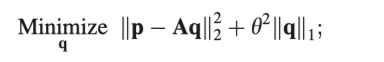# About the square of the two norm of a complex number

Hellow professor
I have a question

such ascvx_begin
variable q(size(A,2)) complex
minimize ( norm(q,1) )
subject to
minimize (sum_square_abs(p-Aq)+lambda*norm(q,1))
cvx_end

or

cvx_begin
variable q(size(A,2)) complex
minimize ( norm(q,1) )
subject to
minimize (square_pos(norm(p-Aq))+lambda*norm(q,1))
cvx_end
which is ok ？
(Where A is a complex number)

Thanks

Each CVX invocation, i…e. code between `cvx_begn` and `cvx_end`may have at most one minimize or maximize statement. Minimize statements are not allowed as constraints (actually, `subject to` is a placebo - it doesn’t do anything,and is only provided for users who wish to use to make their code more understandable). So your program has two minimize statements, which is not allowed by CVX.

``````cvx_begin
variable q(size(A,2)) complex
minimize (sum_square_abs(p-Aq)+lambda*norm(q,1))
cvx_end
``````

should work.

Alternatively, you change the minimize statement to
`minimize (square_pos(norm(p-Aq))+lambda*norm(q,1))`

``````cvx_begin
variable q(size(A,2)) complex
minimize (sum_square_abs(p-Aq))
norm(q,1) <= alpha
cvx_end
``````

which, for a given value of lambda, is equivalent to the original problem for some appropriate value of alpha.

1 Like

Sorry, teacher. I made a mistake in the question, as you did
cvx_begin
variable q(size(A,2)) complex
minimize (sum_square_abs(p-Aq)+lambdanorm(q,1))
cvx_end
or
cvx_begin
variable q(size(A,2)) complex
minimize (square_pos(norm(p-Aq))+lambda
norm(q,1))
cvx_end

My understanding is that this formula means the same thing:
square_pos(norm( p-Aq)) and sum_square_abs(p-Aq).
They can all express the meaning ofAnother problem is that I also sometimes use the following form
cvx_begin
variable q(size(A,2)) complex
minimize ( norm(q,1) )
subject to
norm(ph-Aq)<= lambda;
cvx_end
What is the difference between this formula and the one you suggested to me？
Thank you, my dear teacher

Those are all equivalent formulations, presuming compatible values of alpha and lambda are chosen (these compatible values will not generally be known in advance). Each equivalent formulation will have its own compatible values of alpha and lambda.

BTW, I am not a professor, just an ordinary Joe, as we say in the U.S.A…